# Pascal theorem

(diff) ← Older revision | Latest revision (diff) | Newer revision → (diff)

The opposite sides of a hexagon (cf. Polygon) inscribed in a second-order curve (a conic) intersect in three collinear points (on the Pascal line, see Fig. a). When two adjacent vertices coincide, the line passing through them is understood to be the tangent to the conic at that point. The tangent to a conic drawn at one of the vertices of an inscribed pentagon intersects the side opposite to this vertex at a point on the line passing through the points of intersection of the remaining pairs of non-adjacent sides of the pentagon (see Fig. b).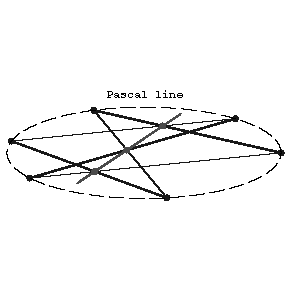Figure: p071780a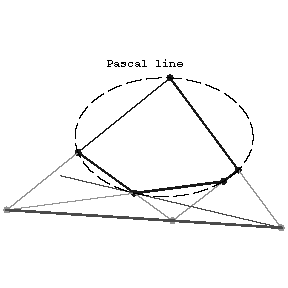Figure: p071780b

If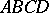is a quadrangle inscribed in a conic, then the points of intersection of the tangents atandwith the sides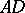and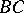, respectively, and the point of intersection of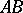and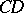are collinear (see Fig. c).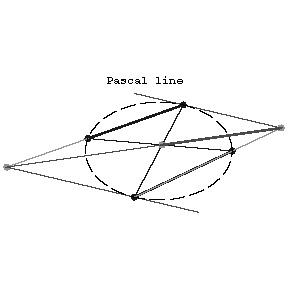Figure: p071780c

The points of intersection of the tangent at the vertices of a triangle inscribed in a conic with the opposite sides are collinear (see Fig. d).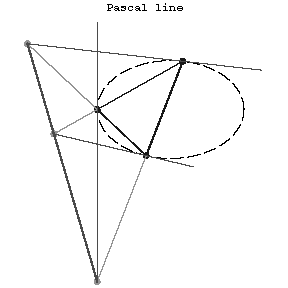Figure: p071780d

The dual to Pascal's theorem is the Brianchon theorem. B. Pascal established his theorem in 1639. The special case of a conic degenerating to a pair of lines was known even in Antiquity (see Pappus axiom).